# Samacheer Kalvi 11th Computer Science Solutions Chapter 8 Iteration and Recursion

Students can Download Computer Science Chapter 8 Iteration and Recursion Questions and Answers, Notes Pdf, Samacheer Kalvi 11th Computer Science Book Solutions Guide Pdf helps you to revise the complete Tamilnadu State Board New Syllabus and score more marks in your examinations.

## Tamilnadu Samacheer Kalvi 11th Computer Science Solutions Chapter 8 Iteration and Recursion

### Samacheer Kalvi 11th Computer Science Iteration and Recursion Text Book Back Questions and Answers

PART – 1
I. Choose The Correct Answer

Question 1.
A loop invariant need not be true ………………….
(a) at the start of the loop
(b) at the start of each iteration
(c) at the end of each iteration
(d) at the start of the algorithm
Answer:
(d) at the start of the algorithm

Question 2.
We wish to cover a chessboard with dominoes,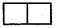the number of black squares and the number of white squares covered by dominoes, respectively, placing a domino can be modeled by ………………….
(a) b : = b + 2
(b) w : = w + 2
(c) b, w : = b + 1, w + 1
(d) b : = w
Answer:
(c) b, w : = b + 1, w + 1

Question 3.
If m x a + n x b is an invariant for the assignment a, b : = a + 8, b + 7, the values of m and n are ………………….
(a) m = 8, n = 7
(b) m = 7, n = – 8
(c) m = 7, n = 8
(d) m = 8, n = – 7
Answer:
(b) m = 7, n = – 8Question 4.
Which of the following is not an invariant of the assignment?
m, n : = m + 2, n + 3
(a) m mod 2
(b) n mod 3
(c) 3 x m – 2 x n
(d) 2 x m – 3 x n
Answer:
(c) 3 x m – 2 x n

Question 5.
If Fibonacci number is defined recursively as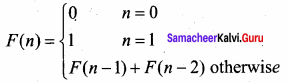to evaluate F(4), how many times F( ) is applied?
(a) 3
(b) 4
(c) 8
(d) 9
Answer:
(d) 9

Question 6.
Using this recursive definition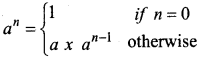how many multiplications are needed to calculate a10?
(a) 11
(b) 10
(c) 9
(d) 8
Answer:
(b) 10

PART – 2
II. Short Answers

Question 1.
What is an invariant?
Answer:
An invariant is a condition that can be relied upon to be true during the execution of a program, or during some portion of it.

Question 2.
Define a loop invariant.
Answer:
In iteration, the loop body is repeatedly executed as long as the loop condition is true. Each time the loop body is executed, the variables are updated. However, there is also a property of the variables which remains unchanged by the execution of the loop body. This unchanging property is called the loop invariant.Question 3.
Does testing the loop condition affect the loop invariant? Why?
Answer:
An invariant is a statement placed in a code segment that is repeated should be true each time the loop execution reaches that point. If an invariant is placed at the beginning of a while or do..while loop whose condition test does not change the state of the computation, then the invariant should also be true at the bottom of the loop.

Question 4.
What is the relationship between loop invariant, loop condition, and the input-output recursively?
Answer:
A loop invariant is a condition [among program variables] that is necessarily true immediately before and immediately after, each iteration of a loop. A loop invariant is some condition that holds for every iteration of the loop.

Question 5.
What is recursive problem-solving?
Answer:
The recursion step breaks the problem into sub-problems of smaller size, assumes solutions for sub-problems are given by recursive calls, and constructs solutions to the given problem.

Question 6.
Define factorial of a natural number recursively.
Answer: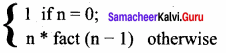Recursive Algorithm:
Fact (n)
– – inputs : n
– – outputs : Fact = n!
if (n = 0) – – base case
1
else
n * fact (n – 1) – – recursive step

PART – 3
III. Explain in Brief

Question 1.
There are 7 tumblers on a table, all standing upside down. You are allowed to turn any 2 tumblers simultaneously in one move. Is it possible to reach a situation when all the tumblers are right side up? (Hint: The parity of the number of upside-down tumblers is invariant.)
Answer:
The answer is No.
The algorithm suggests that we introduce just one variable, namely the number of tumblers that are upside-down call it u.
There are three possible effects of turning two of the tumblers.
Two tumblers that are both sides up are turned upside down. The is represented by
u : = u + 2
Turning two tumblers that are both upsides down has the opposite effect: u decreased by 2.
This is represented by
u: = u – 2
Finally turning two tumblers that are the opposite way up ie. one upside down, the other upside up has no effect on u. It is represented as
u: = u it may be called a skip
The choice of which of these three statements is executed is left unspecified. An invariant of the turning process must therefore be an invariant of the three.

Everything is an invariant skip. So, we can discount skip. We, therefore, seek an invariant of the two assignments u: = u + 2 and u: = u – 2. What does not change if we add or subtract two from u?
The answer is called parity of u. The parity is a boolean value, it is either true or false.
It is true if u Is even (0,2,4…) and it is false if u is odd (1,3,5…).
Let us write even(u) for this boolean quantity as

even(u) |u : = u + 2| = even(u + 2) = even(u) ie. even(u) is an invariant of the assignment u : = u + 2.
even(u) |u : = u – 2| = even(u – 2) = even(u) ie, even(u) is an invariant of the assignment u : = u – 2.

We conclude that, no matter how many times we turn two tumblers over, the parity of the number of upside-down tumblers will not change. If there is an even number at the outset, there will always be an even number; if there is an odd number at the outset, there will always be an odd number. The goal is to repeat the turning process until there is zero upside-down. Zero is an even number. So, the answer to the question is that there must be an even number of upside-down numbers at the outset.Question 2.
A knockout tournament is a series of games. Two players compete in each game; the loser is knocked out (i.e. does not play anymore), the winner carries on. The winner of the tournament is the player that is left after all other players have been knocked out. Suppose there are 1234 players in a tournament. How many games are played before the tournament winner is decided?
Answer: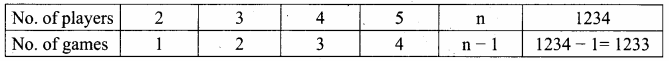On the other hand, let n be the number of games, played and r be the number of players remaining in the tournament.
After every game, r will be reduced by 1.
r → no. of players remaining
n → no. of games played
If r = 2 then n = 1
As n increases, r decreases
n, r : = n + 1, r – 1
n + r = (n + 1) + (r – 1)
= n + 1 + r – 1
= n + r
Therefore n + r is invariant. n + r = 1234 (No. of players initially)
The winner of the tournament is the player that is left after all other players have been knocked out.
After all the games, only one player (winner) is left out.
i. e. n = 1
Put n = 1 in (1)
n + r = 1234 …. (1)
1 + r = 1234
r = 1234 – 1 = 1233
No. of games played = 1233

Question 3.
King Vikramaditya has two magic swords. With one, he can cut off 19 heads of a dragon, but after that, the dragon grows 13 heads. With the other sword, he can cut off 7 heads, but 22 new heads grow. If all heads are cut off, the dragon dies. If the dragon has originally 1000 heads, can it ever die? (Hint: The number of heads mod 3 is invariant.)
Answer:
The dragon never dies.
head(h)
inputs: h is an integer
outputs: h is an integer
h: =1000
while h ≠ 0
if h > = 19
h := h- 19 + 13
else
if h>=7
h := h-7 + 22
else
h := 22;

PART – 4
IV. Explain in Detail

Question 1.
Assume an 8 x 8 chessboard with the usual coloring. “Recoloring” operation changes the color of all squares of a row or a column. You can recolor repeatedly. The goal is to attain just one black square. Show that you cannot achieve the goal.
(Hint: If a row or column has b black squares, it changes by (|(8 – b) – b|).
Answer:
In a chessboard no. of squares in any row or column = 8
Total No. of squares = 8 x 8 = 64
No. of black squares = 32
No. of white squares = 32
Let No. of blacks in a selected recoloring row or column = b
No. of black squares after recoloring operation = 8 – b
Initial state b = 32
Desired final state b = 1
Let us tabulate all the possible outcomes after recoloring a row or column.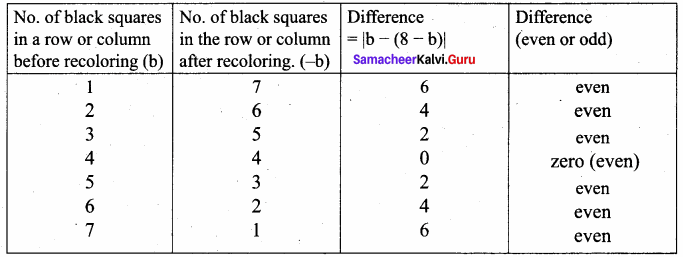The difference is the no. of black squares left out in a row or column after recoloring operation. The difference is even. The difference is invariant. But our goal is one ( 1 is odd) black square, so, it is not possible to attain the goal.

Question 2.
Power can also be defined recursively as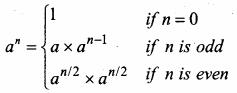Construct a recursive algorithm using this definition. How many multiplications are needed to calculate a10?
Answer:
Power:
power (5, 2) = 5 x 5 = 25
power (x, n) raise x to the power n

Algorithm: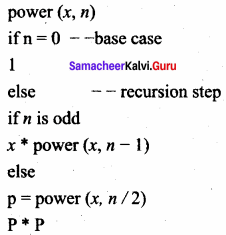To find a10: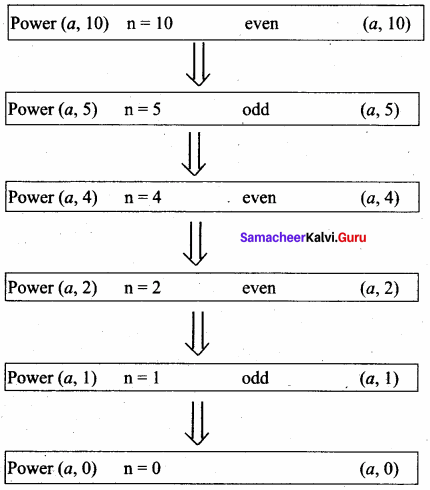Question 3.
A single-square-covered board is a board of 2n x 2n squares in which one square is covered with a single square tile. Show that it is possible to cover this board with triominoes without overlap.
Answer:
The size of the board is 2nn x 2n
Number of squares = 2n x 2n = 4n
Number of squares covered = 1
Number of squares to be covered = 4n – 1
4n – 1 is a multiple of 3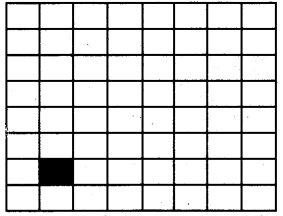Case 1 : n = 1
The size of the board 2 x 2
one triominoe can cover 3 squares without overlap.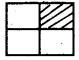We can cover it with one triominoe and solve the problem.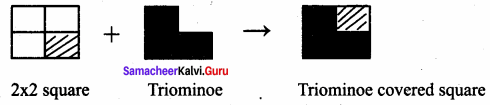Case 2: n ≥ 2
1. place a triominoe at the center of the entire board so as to not cover the covered sub-board.

2. One square in the board is covered by a tile. The board has 4 sub-boards of size 22n – 1 x 22n – 1.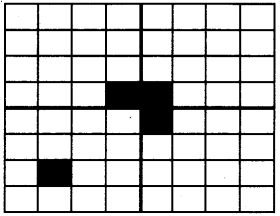Out of 4 sub-boards, one sub-board is a single square covered sub-board.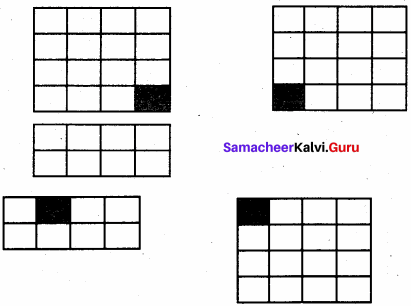One triominoe can cover the remaining three sub-boards into a single square-covered sub-board.
The problem of size n is divided into 4 subproblems of size (n – 1).
Each sub – board has 22n – 1 x 22n – 1 – 1 = 22n – 2 – 1 = 4n – 1 – 1 squares to be covered.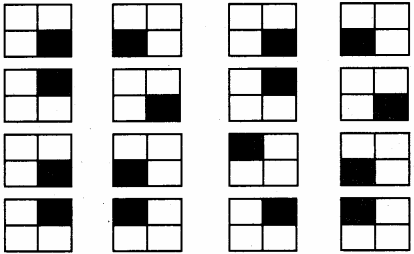4n – 1 – 1 is also a multiple of 3
In this, the 2n x 2n board is reduced to boards of size 2×2 having are square covered. A triominoe can be placed in each of these boards and hence the whole original 2n x 2n. the board is covered with triominoe without overlap.

### Samacheer Kalvi 11th Computer Science Iteration and Recursion Additional Questions and Answers

PART – 1
I. Choose the correct answer

Question 1.
In _________, your body is repeatedly executed as long as the loop condition is true,
a) sequence
b) iteration
c) recursion
d) branching
Answer:
b) iteration

Question 2.
In which year E W Dijkstra was awarded ACM Turing Award?
(a) 1972
(b) 1974
(c) 1970
(d) 1911
Answer:
(a) 1972Question 3.
_________ is the key to constructing and reasoning about iterative algorithms.
a) Loop invariant
b) Loop variant
c) Composition
d) None of these
Answer:
a) Loop invariant

Question 4.
Recursion must have at least ………………… base case.
(a) one
(b) two
(c) three
(d) four
Answer:
(a) one

Question 5.
A recursive solver has _________ cases.
a) three
b) two
c) many
d) no
Answer:
b) two

Question 6.
………………… is the algorithm design techniques to execute the same action repeatedly.
(a) Iteration
(b) Recursion
(c) Both a & b
(d) none of these
Answer:
(c) Both a & b

Question 7.
How many base cases in a recursion?
a) no
b) atleast one
c) only one
d) None of these
Answer:
b) atleast one

Question 8.
The loop invariant need not be true at the …………………
(a) Start of the loop
(b) end of the loop
(c) end of each iteration
(d) middle of algorithm
Answer:
(d) middle of algorithm

Question 9.
Iteration repeats the two steps of evaluating a condition and executing a statement, as long as the condition is _________
a) true
b) false
c) true or false
d) None of these
Answer:
a) true

Question 10.
The input size to a sub-problem is than the input size of the original problem.
(a) equal
(b) smaller
(c) greater
(d) no criteria
Answer:
(b) smaller

Question 11.
When a loop ends,_________
a) the loop invariant is true
b) the termination condition is true
c) Both A and B
d) None of these
Answer:
c) Both A and B

Question 12.
How many cases are needed for recursive solvers?
(a) 2
(b) 3
(c) 4
(d) 5
Answer:
(a) 2Question 13.
To construct a loop, _________
a) Establish the loop invariant at the start of the loop.
b) The loop body should so update the variables as to progress toward the end and maintain the loop invariant, at the same time.
c) When the loop ends, the termination condition and the loop invariant should establish the input-output relation.
d) All the above
Answer:
b) The loop body should so update the variables as to progress toward the end and maintain the loop invariant, at the same time.

Question 14.
Which is the key to construct iterative algorithm …………………
(a) loop invariant
(b) Variable
(c) loop
(d) Recursive
Answer:
(a) loop invariant

Question 15.
Identify the correct statement from the following.
a) When the solver calls a sub-solver, it is known as a recursive call.
b) From the solution to the sub-problem, the solver constructs the solution to the given problem
c) The input size to a sub-problem is smaller than the input size of the original problem.
d) All the above
Answer:
d) All the above

PART – 3
II. Short Answers

Question 1.
Define Iteration.
Answer:
In iteration, the loop body is repeatedly executed as long as the loop condition is true. Each time the loop body is executed, the variables are updated.

Question 2.
What is an invariant?
Answer:
An expression involving variables, which remains unchanged by an assignment to one of these variables, is called an invariant of the assignment.

PART – 3
III. Explain in Brief

Question 1.
What are the constraints to construct a loop?
Answer:
To construct a loop,

• Establish the loop invariant at the start of the loop.
• The loop body should so update the variables as to progress toward the end and maintain the loop invariant, at the same time.
• When the loop ends, the termination condition and the loop invariant should establish the input-output relation.

Question 2.
Write down the steps to be taken to construct a loop.
Answer:

1. Establish the loop invariant at the start of the loop.
2. The loop body should so update the variables as to progress toward the end and maintain the loop invariant, at the same time.
3. When the loop ends, the termination condition and the loop invariant should establish the input-output relation.Question 3.
Write a note on Iteration.
Answer:
Iteration:
In iteration, the loop body is repeatedly executed as long as the loop condition is true. Each time the loop body is executed, the variables are updated. However, there is also a property of the variables which remains unchanged by the execution of the loop body. This unchanging property is called the loop invariant. Loop invariant is the key to construct and to reason about iterative algorithms.

PART – 4
IV. Explain in Detail

Question 1.
Explain Loop invariant with a near diagram.
Answer:
In a loop, if L is an invariant of the loop body B, then L is known as a loop invariant, while C
– – L
B
– – L
The loop invariant is true before the loop body and after the loop body, each time. Since L is true at the start of the first iteration, L is true at the start of the loop also (just before the loop). Since L is true at the end of the last iteration, L is true when the loop ends also (just after the loop). Thus, if L is a loop variant, then it is true at four important points in the algorithm, as annotated in the algorithm.

1. At the start of the loop (just before the loop)
2. at the start of each iteration (before loop body)
3. at the end of each iteration (after loop body)
4. at the end of the loop (just after the loop)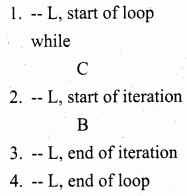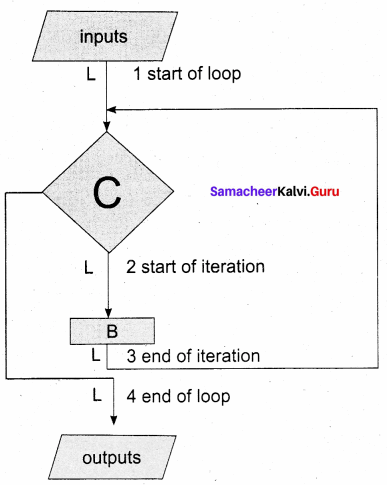Question 2.
Explain recursive problem-solving.
Answer:
To solve a problem recursively, the solver reduces the problem to subproblems, and calls another instance of the solver, known as sub-solver, to solve the sub – problem. The input size to a sub-problem is smaller than the input size of the original problem. When the solver calls a sub-solver, it is known as a recursive call. The magic of recursion allows the solver to assume that the sub-solver (recursive call) outputs the solution to the sub-problem. Then, from the solution to the sub-problem, the solver constructs the solution to the given problem. As the sub-solvers go on reducing the problem into subproblems of smaller sizes, eventually the sub-problem becomes small enough to be solved directly, without recursion. Therefore, a recursive solver has two cases:
1. Base case:
The problem size is small enough to be solved directly. Output the solution. There must be at least one base case.

2. Recursion step:
The problem size is not small enough. Deconstruct the problem into a sub-problem, strictly smaller in size than the given problem. Call a sub-solver to solve the sub-problem. Assume that the sub-solver outputs the solution to the sub-problem. Construct the solution to the given problem. This outline of the recursive problem-solving technique is shown below.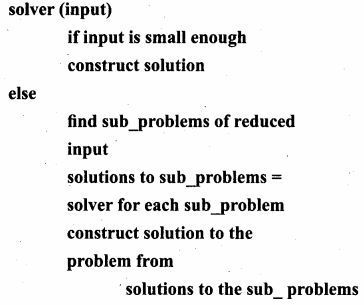Whenever we solve a problem using recursion, we have to ensure these two cases: In the recursion step, the size of the input to the recursive call is strictly smaller than the size of the given input, and there is at least one base case.

Question 3.
Give an example for loop invariant.
Answer:
The loop invariant is true in four crucial points in a loop. Using the loop invariant, we can construct the loop and reason about the properties of the variables at these points.
Example:
Design an iterative algorithm to compute an. Let us name the algorithm power(a, n). For example,
power(10, 4) = 10000
power (5, 3) = 125
power (2, 5) = 32
Algorithm power(a, n) computes an by multiplying a cumulatively n times,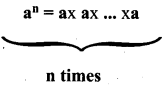The specification and the loop invariant are shown as comments.
power (a, n)
– – inputs: n is a positive integer
– – outputs: p = an
p, i : = 1, 0
while i ≠ n
– – loop invariant: p = ai
p, i : = p x a, i + 1
The step-by-step execution of power (2, 5) is shown in Table. Each row shows the values of the two variables p and i at the end of an iteration, and how they are calculated. We see that p = ai is true at the start of the loop, and remains true in each row. Therefore, it is a loop invariant.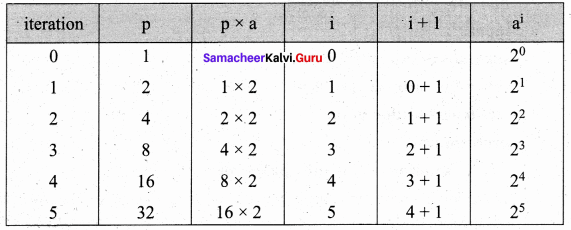When the loop ends, p = ai is still true, but i = 5. Therefore, p = a5. In general, when the loop ends, p = an . Thus, we have verified that power(a, n) satisfies its specification.

Question 4.
There are 6 equally spaced trees and 6 sparrows sitting on these trees, one sparrow on each tree. If a sparrow flies from one tree to another, then at the same time, another sparrow flies from its tree to some other tree the same distance away, but in the opposite direction. Is it possible for all the sparrows to gather on one tree?
Answer:
Let us index the trees from 1 to 6. The index of a sparrow is the index of the tree it is currently sitting on. A pair of sparrows flying can be modeled as an iterative step of a loop. When a sparrow at tree i flies to the tree i + d, another sparrow at tree j flies to tree j – d. Thus, after each iterative step, the sum S of the indices of the sparrows remains invariant. Moreover, a loop invariant is true at the start and at the end of the loop.
At the start of the loop, the value of the invariant is
S = 1 + 2 + 3 + 4 + 5 + 6 = 21
When the loop ends, the loop invariant has the same value. However, when the loop ends, if all the sparrows were on the same tree, say k, then S = 6k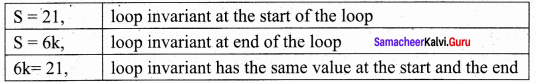It is not possible – 21 is not a multiple of 6. The desired final values of the sparrow indices are not possible with the loop invariant. Therefore, all the sparrows cannot gather on one tree.

Question 5.
Customers are waiting in a line at a counter. The man at the counter wants to know how many customers are waiting in the line.
Answer:
Customers are waiting in a line at a counter. The man at the counter wants to know how many customers are waiting in the line.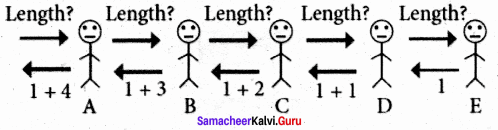Instead of counting the length himself, he asks customer A for the length of the line with him at the head, customer A asks customer B for the length of the line with customer B at the head, and so on. When the query reaches the last customer in the line, E, since there is no one behind him, he replies 1 to D who asked him. D replies 1 + 1 = 2 to C, C replies 1 + 2 = 3 to B, B replies 1 + 3 = 4 to A, and A replies 1 + 4 = 5 to the man in the counter.

Question 6.
Design a recursive algorithm to compute an. We constructed an iterative algorithm to compute an in Example 8.5. an can be defined recursively as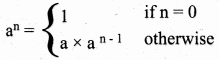Answer:
The recursive definition can be expressed as a recursive solver for computing power(a, n).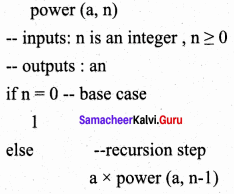The recursive process resulting from power(2, 5)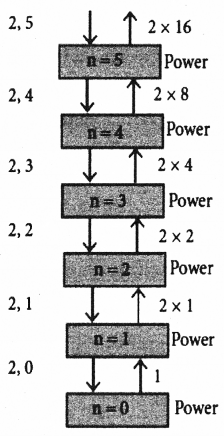power (2, 5)
= 2 x power (2, 4)
= 2 x 2 x power (2, 3)
= 2 x 2 x 2 x power (2, 2)
= 2 x 2 x 2 x 2 x power (2, 1)
= 2 x 2 x 2 x 2 x 2 x power (2, 0) = 2 x 2 x 2 x 2 x 2 x 1
= 2 x 2 x 2 x 2 x 2 = 2 x 2 x 2 x 4 = 2 x 2 x 8 = 2 x 16 = 32